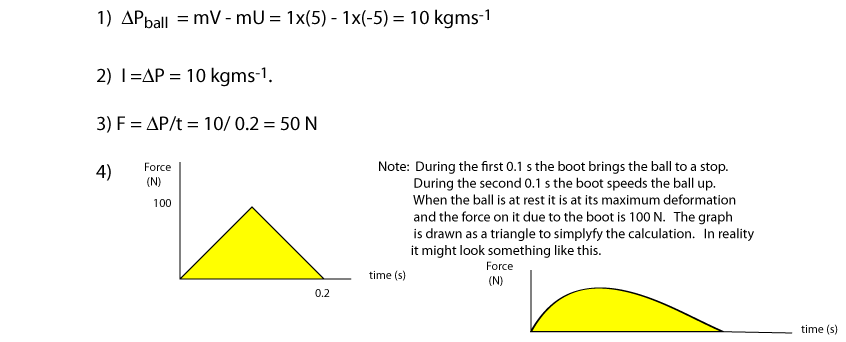Aids to Understanding

Vectorial Issues

Classifying physical quantities as vectors is extremely useful but only if we think vectorially. For example, velocity, acceleration and momentum are vectors and have both magnitude and direction. Now if these quantities change it should be clear that it can be either the magnitude or direction of the quantity that has changed. You will be tempted to think of a change as either an increase or decrease, and when working with scalars, this is fine, however you should avoid thinking in in terms of increasing and decreasing when working with vectors. One example is the motion of the Earth round the sun. The velocity of the Earth is changing by virtue of a changing direction and not due to an increasing or decreasing magnitude. This point will be clearer when discussing certain momentum contexts.

Elastic and Inelastic collisions

The elasticity of a collision depends on the ability of the colliding objects to restore themselves to their original shapes after the collision. Snooker balls can clearly do this so more often than not a collision between two of them tends to be elastic (Kinetic energy is conserved). However if two cars crash and crumple up then energy of the collision is used deforming the cars and the collision is inelastic.
If two bodies join and move off after a collision kinetic energy is never conserved but can you prove this? Momentum is always conserved because each colliding body exerts an equal and opposite force on each other.

Momentum Problem

Look at the problem under the heading 5) Colliding bodies. The velocity of trolley B was chosen to be 2.0 ms-1, and was chosen arbitralily. If we change the rubber stoppers on each car to be more or less rubbery then this speed will change. Suppose the velocity of car B after the collision is 1.5 ms-1. Repeat the problem now.

Conservation of Momentum and the pendulum

a) Suppose an arrow of mass 200 g and travelling at 100 ms-1 hits and imbeds itself into a stationary 10 kg block of wood hanging from a roof. See diagram below. To what height will the block rise?

b) Suppose a ball of mass 200 g and travelling at 100 ms-1 hits and rebounds from a stationary 10 kg block of wood hanging from a roof. To what height will the block rise, if the block rebounds at -10ms-1?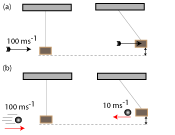Impulse and Work

The following example should clear up the difference between these two concepts. Example: Suppose a force of 6 N pulls a 3kg trolley for 10 s. During this time suppose it travels 20 m and its initial velocity is 2ms-1
i) Calculate the final momentum of the trolley
ii) Calculate the final kinetic energy of the trolley.
iii) If the force is constant, draw a force-time graph and a force - displacement graph for the trolley and indicate the physical quantities that the areas under these graphs mean
Which area above represents a vector.

i) Ft = DP = mv - mu
mv = mu + Ft
3x(-2) + 6x10 = 54 Kgms-1
ii) Fd = DEk = 1/2mv2 - 1/2mu2
1/2mv2 = Fs + 1/2mu2
= 90 + 6 = 96 J.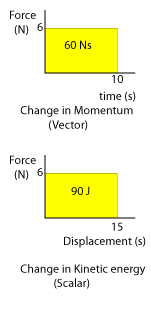Cumulative problem 1

Forces are fundamental concepts in physics and velocity-time generated by data loggers and computer programmes allow us to find the value of contact forces that act over very small time intervals. The concept of Impulse helps us to this end.

Example:

A 250g ball is allowed to fall from a height of 1.25 m and allowed to bounce once. The idealised velocity-time graph for the ball's motion is shown below.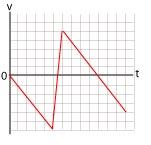Your job is to label the axes and draw an force-time graph for the ball's motion for the time the ball is in contact with the ground. The answer is provided below, but try to do it yourself before you look at it. The successful attempt indicates clear understanding of much of the previous work.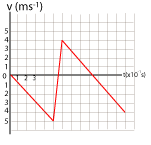Cumulative problem 2

Two bodies of equal mass fall to the ground from the same height without bouncing. The only difference between these bodies is that one of them is soft and the other is hard.
i) Draw the shapes of the v-t graph for each body, until the upwards force on the body due to the ground (FBG) equals the body's weight.
ii) Draw the shapes of the F-t graph for each ball, where t is the time the body is in contact with the ground whilst the upwards force due to the ground is greater than its weight.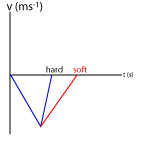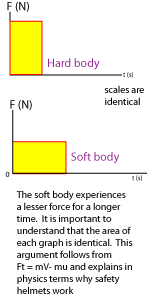Idealised force-time graphs

A falling ball reaching ground will not experience a constant force on contact with the ground. The force from the ground gradually increases until the ball is at rest, then the force decreases until the ball eventually rises. The force-time, acceleration-time and velocity-time graphs for the ball are shown below. The area under f-t graph is positive so the change in momentum and acceleration must also be positive. Note when the force on the ball is a maximum, so is the acceleration of the ball. At this point the ball is at its maxiumum deformation and its velocity is zero (ready to recoil).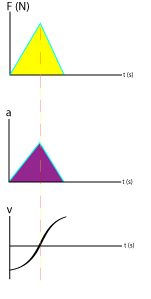## 1) Momentum

Momentum is a useful quantity because it is a conserved quantity and as such can be used to predict the motion of bodies after a collision. Momentum is a vector and is defined as a product of mass x velocity. If a car has a velocity of 15 ms-1 and mass of 1500 kg, then its momentum is in the same direction as its velocity and has a magnitude of 22500 kgms-1. The symbol for momentum is P, ie P=mv. In the diagram below put the bodies in order of increasing momentum. You are invited to estimate the mass of each body.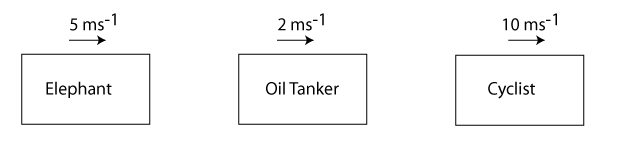The order should be oiltanker, elephant and cyclist.

## 2) Conservation of Momentum and Newton's Third law of motion

Momentum is useful to us because it allows us to make predictions about a bodY's motion after it has collided with another. When two bodies, A and B collide they exert a force on each other. From Newton's third Law of Motion we know the forces are equal in magnitude but opposite in direction, ie FAB = -FBA. The bodies, can change direction during the collision or change their motion in other ways, but one thing we know for sure, because they exert opposite and equal forces on each other, the total momentum of the bodies before the collision equals the total momentum of the bodies after the collision. This is known as the Law of Conservation of Momentum. The following analysis shows how the Conservation of Momentum follows from Newton's Third law. You may have found the same result empirically by carefully measuring the masses and initial and final velocities of colliding bodies.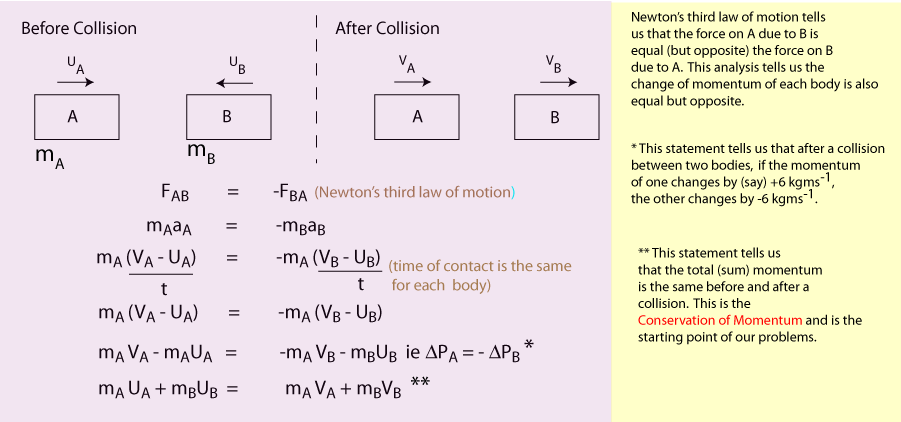## 3) Elastic Collisions and Kinetic energy

Collisions can be classified as elastic or inelastic. In elastic collisions the total kinetic energy before a collision equals the kinetic energy after the collision and we say kinetic energy is connserved. Super bouncy balls and colliding billiard balls deform during a collision but quickly restore themselves to their original shapes, with no deformation. During the collision kinetic energy is changed to elastic potential energy and then back to kinetic as they move off. In these collisions the interacting forces do virtually no work against friction and therefore there are no energy losses and no (ideally) heat energy or sound energy produced during the collision. The diagram shows two colliding vehicles with elastic bands attached.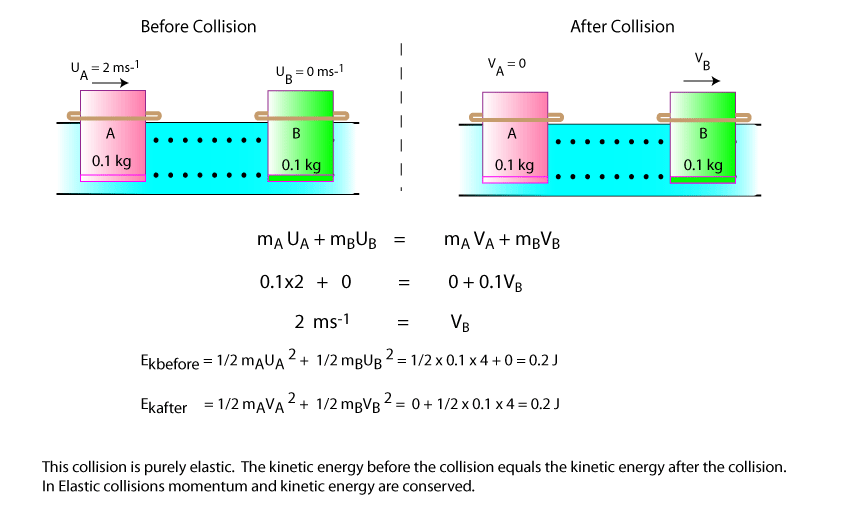## 4) Inelastic Collisions and Kinetic energy.

If, during a collision between two bodies, deformation of some sort occurs then heat energy will be produced (and sound). These collisions are inelastic and momentum alone is conserved. In the diagram below vehicle A has a pin attached to it and vehicle B has a cork attached to it. During the coupling (deformation) the frictional force on the pin does work and thus some of the kinetic energy of vehicle A has been changed to heat during the collision. This means that, in this type of collision, kinetic energy is not conserved. Newton's third law still holds so momentum is still conserved.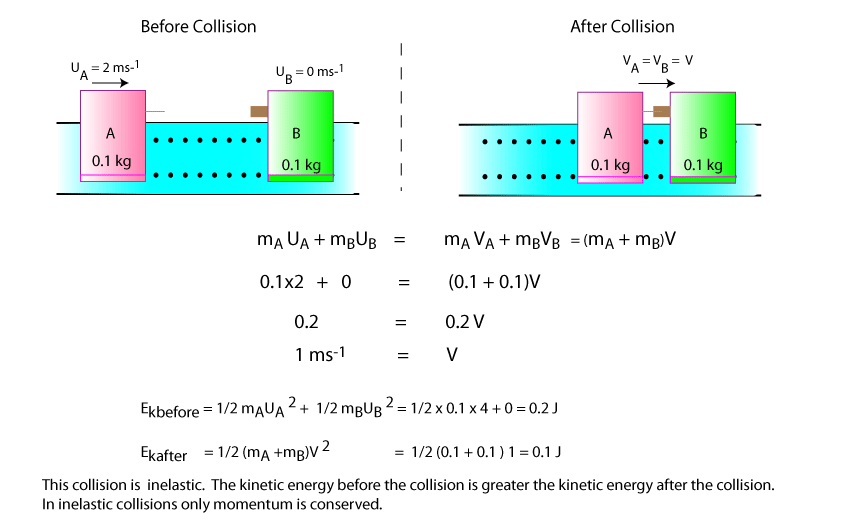## 5) Momentum Calculation (Colliding bodies)

In this problem two vehicles approach each other and rebound. It is important we remember to think vectorially and assign the correct sign to the velocity vectors. Motion to the left is, as usual, taken as negative. Study the diagram carefully and attempt the following...
(1) Find the velocity of A after the collision.
(2) Verify that DPA = - DPB ie Show the change in momentum of A is equal (but opposite) the mometum of B.
(3) Is the collision elastic or inelastic?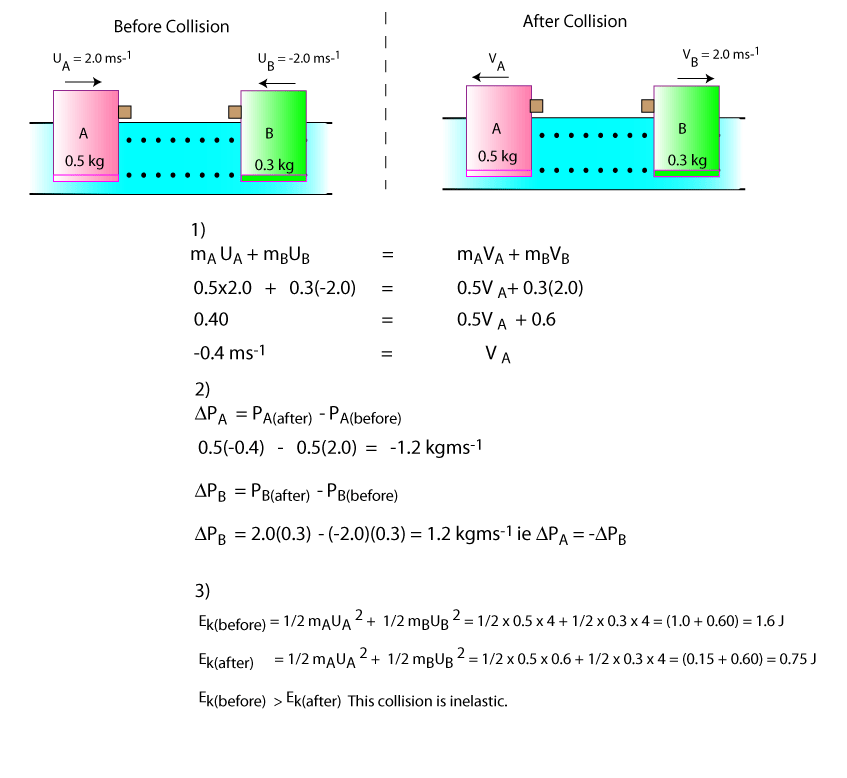## 6) Momentum Calculation (Explosions)

The Conservation of Momentum can also be applied to bodies at rest and then push each apart. The usual context for this can be a cannonball leaving a cannon or two ice skaters at rest pushing each other in different directions. The main point to remember is that the total initial momentum of the bodies is zero therefore the total final momentum of the bodies must also be zero. Study the diagram carefully and attempt the following...
(1) Find the velocity of A after the collision.
(2) Verify that DPA = - DPB ie Show the change in momentum of A is equal (but opposite) the mometum of B.
(3) Verify Newton's Third law of Motion, ie show that FAB = -FBA

You may assume the time of contact is 0.01s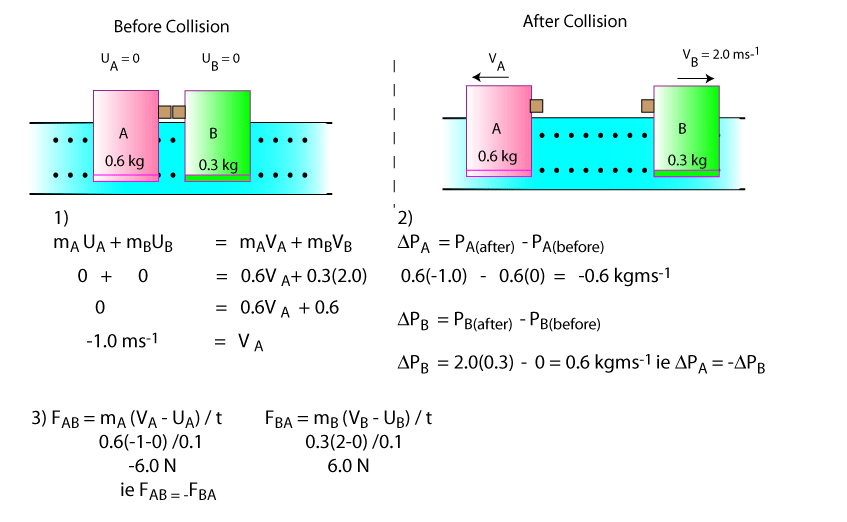## 7) Impulse

If a force is acting on a body for a time t, then we say the Impulse, I, of the force on the body is equal to force x time, ie I = Ft (Ns). Impulse is a vector and give that F = m(v-u)/t, it should be clear that Ft = mv - mu or I = DP. Since masses and speeds are relatively easy to measure and electronic timers can be used to measure short time intervals, this relationship allows us to find the force acting on bodies over short time intervals. The diagram below illustrates how to find the force exterted on a ball due to a cue Fbc. Use the fact that impulse equals change in momentum to verify that Fbc = 11.9 N.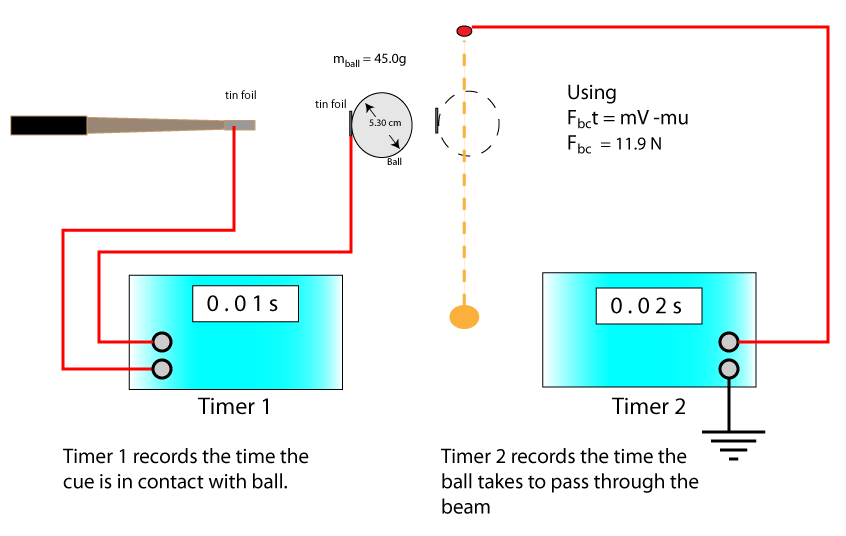## 8) Impulse Calculations

A regular context for impulse in this course is the motorcyclist's helmet. For example, how do we explain the fact that a padded helmet is safer than a non-padded helmet or indeed none at all? To give a proper explanation of some physical phenomenon we must refer to the appropriate physics principle that explains it. To explain why padded helmets prevent injury we state that impulse equals change in momentum (I = DP) and then state Ft = mV-mU. The following example illustrates the usefulness of I = DP.
Example: Suppose two eggs of mass 60 g fall 1 m to the ground and come to rest. One egg is covered in cotton wool of neglible mass. Suppose the egg shell will crack if it experiences a force of 20 N.
1. Calculate DP the change in momentum of each egg
2. What impulse did the ground exert on each egg
3. Suppose the egg with no cotton wool came to rest in 0.02 s and the egg in cotton wool came to rest on 0.5s
4. Calculate, FEG the force exerted on each egg by the ground and state which egg, if any, crack.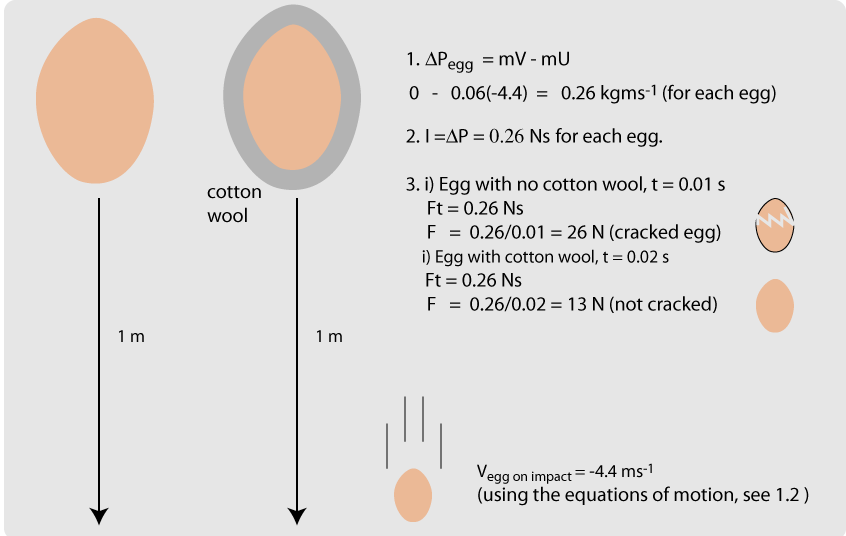Note, DP for each egg was the same, it was the impact time that differed. The impact time for the egg in cotton wool was greater than that for the other egg. Using Ft = 0.26 Ns for each egg showed that the longer the impact time, the less the impact force. You should now be able to explain why crash helmets and padded clothes help protect their wearers.#

#### Force-time graphs

Suppose we were asked to draw a force-time graph for each egg above. If we assumed the force of impact was constant during the impact then the graphs would look like this. Note, the yellow area under the graph is the impulse and is the same for each graph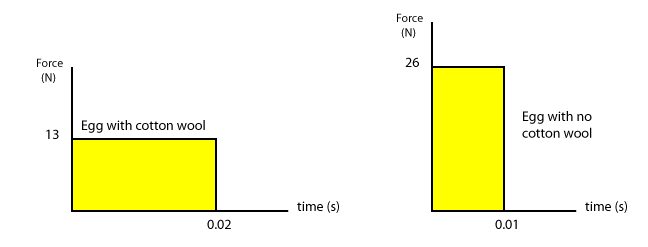In general though, the force on the body is not constant. For example suppose a footballer kicks a 1 kg ball. If the ball was travelling towards the player with a velocity of -5 ms-1 and it leaves his boot travelling in the opposite direction at 5 ms-1, then
1. Calculate the change in momentum of the ball
2. What impulse did the foot exert on the ball
3. Calculate the average force exerted on the ball (assume the ball was in contact with his foot for 0.2 s)
4. Draw a simplified force-time graph for the ball
5.